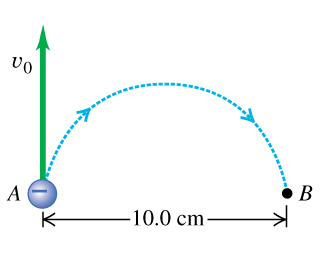# Problem: An electron at point A in the figure (Figure 1) has a speed v0 of 1.60×106 m/s. 1. Find the direction of the magnetic field that will cause the electron to follow the semicircular path from A to B.2 .Find the magnitude of the magnetic field that will cause the electron to follow the semicircular path from A to B.3. Find the time required for the electron to move from A to B.4. Find the direction of the magnetic field, that would be needed if the particle were a proton instead of an electron?5. Find the magnitude of the magnetic field, that would be needed if the particle were a proton instead of an electron?

###### FREE Expert Solution

1.

Using the right-hand rule, place your right hand in the direction of the moving electron and curl your fingers towards the direction of the magnetic field.

95% (492 ratings)###### Problem DetailsAn electron at point A in the figure (Figure 1) has a speed v0 of 1.60×106 m/s.

1. Find the direction of the magnetic field that will cause the electron to follow the semicircular path from A to B.

2 .Find the magnitude of the magnetic field that will cause the electron to follow the semicircular path from A to B.

3. Find the time required for the electron to move from A to B.

4. Find the direction of the magnetic field, that would be needed if the particle were a proton instead of an electron?

5. Find the magnitude of the magnetic field, that would be needed if the particle were a proton instead of an electron?